forecast

Forecast vector error-correction (VEC) model responses

Description

example

Y = forecast(Mdl,numperiods,Y0) returns a path of minimum mean squared error (MMSE) forecasts (Y) over the length numperiods forecast horizon using the fully specified VEC(p – 1) model Mdl. The forecasted responses represent the continuation of the presample data Y0.

example

Y = forecast(Mdl,numperiods,Y0,Name,Value) uses additional options specified by one or more name-value arguments. For example, 'X',X,'YF',YF specifies X as future exogenous predictor data for the regression component and YF as future response data for conditional forecasting.

example

[Y,YMSE] = forecast(___) returns the corresponding mean squared error (MSE) of each forecasted response using any of the input arguments in the previous syntaxes.

Examples

collapse all

Consider a VEC model for the following seven macroeconomic series. Then, fit the model to the data and forecast responses 12 quarters into the future.

• Gross domestic product (GDP)

• GDP implicit price deflator

• Paid compensation of employees

• Nonfarm business sector hours of all persons

• Effective federal funds rate

• Personal consumption expenditures

• Gross private domestic investment

Suppose that a cointegrating rank of 4 and one short-run term are appropriate, that is, consider a VEC(1) model.

For more information on the data set and variables, enter Description at the command line.

Determine whether the data needs to be preprocessed by plotting the series on separate plots.

figure;
subplot(2,2,1)
plot(FRED.Time,FRED.GDP);
title('Gross Domestic Product');
ylabel('Index');
xlabel('Date');
subplot(2,2,2)
plot(FRED.Time,FRED.GDPDEF);
title('GDP Deflator');
ylabel('Index');
xlabel('Date');
subplot(2,2,3)
plot(FRED.Time,FRED.COE);
title('Paid Compensation of Employees');
ylabel('Billions of \$');
xlabel('Date');
subplot(2,2,4)
plot(FRED.Time,FRED.HOANBS);
ylabel('Index');
xlabel('Date');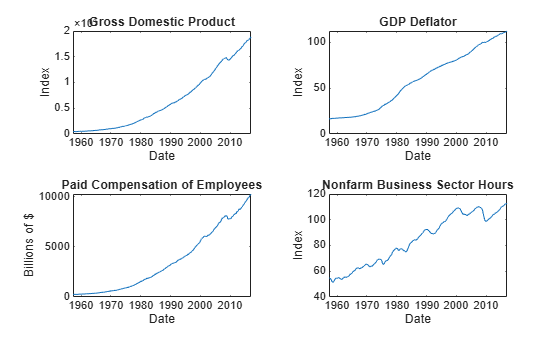figure;
subplot(2,2,1)
plot(FRED.Time,FRED.FEDFUNDS);
title('Federal Funds Rate');
ylabel('Percent');
xlabel('Date');
subplot(2,2,2)
plot(FRED.Time,FRED.PCEC);
title('Consumption Expenditures');
ylabel('Billions of \$');
xlabel('Date');
subplot(2,2,3)
plot(FRED.Time,FRED.GPDI);
title('Gross Private Domestic Investment');
ylabel('Billions of \$');
xlabel('Date');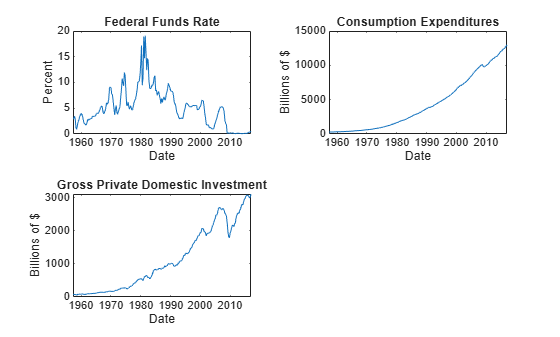Stabilize all series, except the federal funds rate, by applying the log transform. Scale the resulting series by 100 so that all series are on the same scale.

FRED.GDP = 100*log(FRED.GDP);
FRED.GDPDEF = 100*log(FRED.GDPDEF);
FRED.COE = 100*log(FRED.COE);
FRED.HOANBS = 100*log(FRED.HOANBS);
FRED.PCEC = 100*log(FRED.PCEC);
FRED.GPDI = 100*log(FRED.GPDI);

Create a VEC(1) model using the shorthand syntax. Specify the variable names.

Mdl = vecm(7,4,1);
Mdl.SeriesNames = FRED.Properties.VariableNames;

Mdl is a vecm model object. All properties containing NaN values correspond to parameters to be estimated given data.

Estimate the model using the entire data set and the default options.

EstMdl = estimate(Mdl,FRED.Variables)
EstMdl =
vecm with properties:

Description: "7-Dimensional Rank = 4 VEC(1) Model"
SeriesNames: "GDP"  "GDPDEF"  "COE"  ... and 4 more
NumSeries: 7
Rank: 4
P: 2
Constant: [14.1329 8.77841 -7.20359 ... and 4 more]'
Cointegration: [7×4 matrix]
Impact: [7×7 matrix]
CointegrationConstant: [-28.6082 109.555 -77.0912 ... and 1 more]'
CointegrationTrend: [4×1 vector of zeros]
ShortRun: {7×7 matrix} at lag 
Trend: [7×1 vector of zeros]
Beta: [7×0 matrix]
Covariance: [7×7 matrix]

EstMdl is an estimated vecm model object. It is fully specified because all parameters have known values. By default, estimate imposes the constraints of the H1 Johansen VEC model form by removing the cointegrating trend and linear trend terms from the model. Parameter exclusion from estimation is equivalent to imposing equality constraints to zero.

Forecast responses from the estimated model over a three-year horizon. Specify the entire data set as presample observations.

numperiods = 12;
Y0 = FRED.Variables;
Y = forecast(EstMdl,numperiods,Y0);

Y is a 12-by-7 matrix of forecasted responses. Rows correspond to the forecast horizon, and columns correspond to the variables in EstMdl.SeriesNames.

Plot the forecasted responses and the last 50 true responses.

fh = dateshift(FRED.Time(end),'end','quarter',1:12);

figure;
subplot(2,2,1)
h1 = plot(FRED.Time((end-49):end),FRED.GDP((end-49):end));
hold on
h2 = plot(fh,Y(:,1));
title('Gross Domestic Product');
ylabel('Index (scaled)');
xlabel('Date');
h = gca;
fill([FRED.Time(end) fh([end end]) FRED.Time(end)],h.YLim([1 1 2 2]),'k',...
'FaceAlpha',0.1,'EdgeColor','none');
legend([h1 h2],'True','Forecast','Location','Best')
hold off
subplot(2,2,2)
h1 = plot(FRED.Time((end-49):end),FRED.GDPDEF((end-49):end));
hold on
h2 = plot(fh,Y(:,2));
title('GDP Deflator');
ylabel('Index (scaled)');
xlabel('Date');
h = gca;
fill([FRED.Time(end) fh([end end]) FRED.Time(end)],h.YLim([1 1 2 2]),'k',...
'FaceAlpha',0.1,'EdgeColor','none');
legend([h1 h2],'True','Forecast','Location','Best')
hold off
subplot(2,2,3)
h1 = plot(FRED.Time((end-49):end),FRED.COE((end-49):end));
hold on
h2 = plot(fh,Y(:,3));
title('Paid Compensation of Employees');
ylabel('Billions of \$ (scaled)');
xlabel('Date');
h = gca;
fill([FRED.Time(end) fh([end end]) FRED.Time(end)],h.YLim([1 1 2 2]),'k',...
'FaceAlpha',0.1,'EdgeColor','none');
legend([h1 h2],'True','Forecast','Location','Best')
hold off
subplot(2,2,4)
h1 = plot(FRED.Time((end-49):end),FRED.HOANBS((end-49):end));
hold on
h2 = plot(fh,Y(:,4));
ylabel('Index (scaled)');
xlabel('Date');
h = gca;
fill([FRED.Time(end) fh([end end]) FRED.Time(end)],h.YLim([1 1 2 2]),'k',...
'FaceAlpha',0.1,'EdgeColor','none');
legend([h1 h2],'True','Forecast','Location','Best')
hold offfigure;
subplot(2,2,1)
h1 = plot(FRED.Time((end-49):end),FRED.FEDFUNDS((end-49):end));
hold on
h2 = plot(fh,Y(:,5));
title('Federal Funds Rate');
ylabel('Percent');
xlabel('Date');
h = gca;
fill([FRED.Time(end) fh([end end]) FRED.Time(end)],h.YLim([1 1 2 2]),'k',...
'FaceAlpha',0.1,'EdgeColor','none');
legend([h1 h2],'True','Forecast','Location','Best')
hold off
subplot(2,2,2)
h1 = plot(FRED.Time((end-49):end),FRED.PCEC((end-49):end));
hold on
h2 = plot(fh,Y(:,6));
title('Consumption Expenditures');
ylabel('Billions of \$ (scaled)');
xlabel('Date');
h = gca;
fill([FRED.Time(end) fh([end end]) FRED.Time(end)],h.YLim([1 1 2 2]),'k',...
'FaceAlpha',0.1,'EdgeColor','none');
legend([h1 h2],'True','Forecast','Location','Best')
hold off
subplot(2,2,3)
h1 = plot(FRED.Time((end-49):end),FRED.GPDI((end-49):end));
hold on
h2 = plot(fh,Y(:,7));
title('Gross Private Domestic Investment');
ylabel('Billions of \$ (scaled)');
xlabel('Date');
h = gca;
fill([FRED.Time(end) fh([end end]) FRED.Time(end)],h.YLim([1 1 2 2]),'k',...
'FaceAlpha',0.1,'EdgeColor','none');
legend([h1 h2],'True','Forecast','Location','Best')
hold off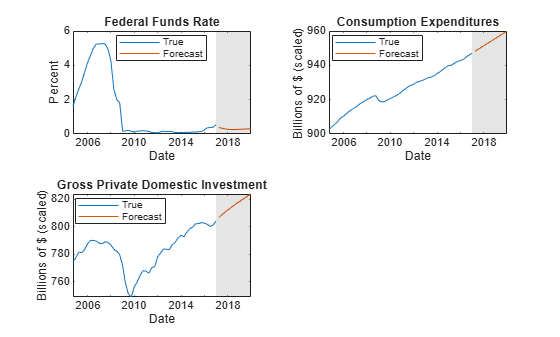Consider the model and data in Forecast Unconditional Response Series from VEC Model.

Load the Data_USEconVECModel data set and preprocess the data.

FRED.GDP = 100*log(FRED.GDP);
FRED.GDPDEF = 100*log(FRED.GDPDEF);
FRED.COE = 100*log(FRED.COE);
FRED.HOANBS = 100*log(FRED.HOANBS);
FRED.PCEC = 100*log(FRED.PCEC);
FRED.GPDI = 100*log(FRED.GPDI);

The Data_Recessions data set contains the beginning and ending serial dates of recessions. Load the data set. Convert the matrix of date serial numbers to a datetime array.

dtrec = datetime(Recessions,'ConvertFrom','datenum');

Create a dummy variable that identifies periods in which the U.S. was in a recession or worse. Specifically, the variable should be 1 if FRED.Time occurs during a recession, and 0 otherwise.

isin = @(x)(any(dtrec(:,1) <= x & x <= dtrec(:,2)));
isrecession = double(arrayfun(isin,FRED.Time));

Create a VEC(1) model using the shorthand syntax. Assume that the appropriate cointegration rank is 4. You do not have to specify the presence of a regression component when creating the model. Specify the variable names.

Mdl = vecm(7,4,1);
Mdl.SeriesNames = FRED.Properties.VariableNames;

Estimate the model using all but the last three years of data. Specify the predictor identifying whether the observation was measured during a recession.

bfh = FRED.Time(end) - years(3);
estIdx = FRED.Time < bfh;
EstMdl = estimate(Mdl,FRED{estIdx,:},'X',isrecession(estIdx));

Forecast a path of quarterly responses three years into the future.

Y0 = FRED{estIdx,:};
Y = forecast(EstMdl,12,Y0,'X',isrecession(~estIdx));

Y is a 12-by-7 matrix of simulated responses. Rows correspond to the forecast horizon, and columns correspond to the variables in EstMdl.SeriesNames.

Plot the forecasted responses and the last 40 true responses.

figure;
subplot(2,2,1)
h1 = plot(FRED.Time((end-39):end),FRED.GDP((end-39):end));
hold on
h2 = plot(FRED.Time(~estIdx),Y(:,1));
title('Gross Domestic Product');
ylabel('Index (scaled)');
xlabel('Date');
h = gca;
fill([bfh h.XLim([2 2]) bfh],h.YLim([1 1 2 2]),'k',...
'FaceAlpha',0.1,'EdgeColor','none');
legend([h1 h2],'True','Forecast','Location','Best')
hold off
subplot(2,2,2)
h1 = plot(FRED.Time((end-39):end),FRED.GDPDEF((end-39):end));
hold on
h2 = plot(FRED.Time(~estIdx),Y(:,2));
title('GDP Deflator');
ylabel('Index (scaled)');
xlabel('Date');
h = gca;
fill([bfh h.XLim([2 2]) bfh],h.YLim([1 1 2 2]),'k',...
'FaceAlpha',0.1,'EdgeColor','none');
legend([h1 h2],'True','Forecast','Location','Best')
hold off
subplot(2,2,3)
h1 = plot(FRED.Time((end-39):end),FRED.COE((end-39):end));
hold on
h2 = plot(FRED.Time(~estIdx),Y(:,3));
title('Paid Compensation of Employees');
ylabel('Billions of \$ (scaled)');
xlabel('Date');
h = gca;
fill([bfh h.XLim([2 2]) bfh],h.YLim([1 1 2 2]),'k',...
'FaceAlpha',0.1,'EdgeColor','none');
legend([h1 h2],'True','Forecast','Location','Best')
hold off
subplot(2,2,4)
h1 = plot(FRED.Time((end-39):end),FRED.HOANBS((end-39):end));
hold on
h2 = plot(FRED.Time(~estIdx),Y(:,4));
ylabel('Index (scaled)');
xlabel('Date');
h = gca;
fill([bfh h.XLim([2 2]) bfh],h.YLim([1 1 2 2]),'k',...
'FaceAlpha',0.1,'EdgeColor','none');
legend([h1 h2],'True','Forecast','Location','Best')
hold off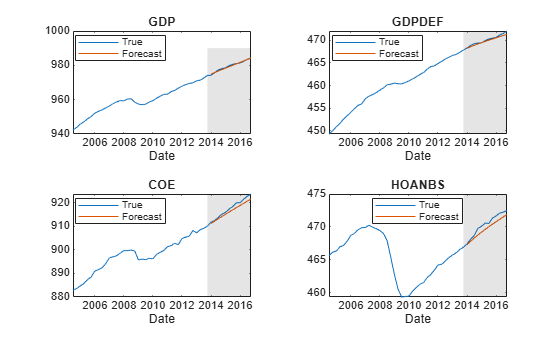figure;
subplot(2,2,1)
h1 = plot(FRED.Time((end-39):end),FRED.FEDFUNDS((end-39):end));
hold on
h2 = plot(FRED.Time(~estIdx),Y(:,5));
title('Federal Funds Rate');
ylabel('Percent');
xlabel('Date');
h = gca;
fill([bfh h.XLim([2 2]) bfh],h.YLim([1 1 2 2]),'k',...
'FaceAlpha',0.1,'EdgeColor','none');
legend([h1 h2],'True','Forecast','Location','Best')
hold off
subplot(2,2,2)
h1 = plot(FRED.Time((end-39):end),FRED.PCEC((end-39):end));
hold on
h2 = plot(FRED.Time(~estIdx),Y(:,6));
title('Consumption Expenditures');
ylabel('Billions of \$ (scaled)');
xlabel('Date');
h = gca;
fill([bfh h.XLim([2 2]) bfh],h.YLim([1 1 2 2]),'k',...
'FaceAlpha',0.1,'EdgeColor','none');
legend([h1 h2],'True','Forecast','Location','Best')
hold off
subplot(2,2,3)
h1 = plot(FRED.Time((end-39):end),FRED.GPDI((end-39):end));
hold on
h2 = plot(FRED.Time(~estIdx),Y(:,7));
title('Gross Private Domestic Investment');
ylabel('Billions of \$ (scaled)');
xlabel('Date');
h = gca;
fill([bfh h.XLim([2 2]) bfh],h.YLim([1 1 2 2]),'k',...
'FaceAlpha',0.1,'EdgeColor','none');
legend([h1 h2],'True','Forecast','Location','Best')
hold off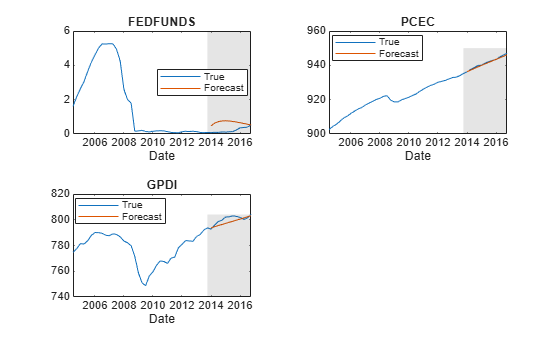Analyze forecast accuracy using forecast intervals over a three-year horizon. This example follows from Forecast Unconditional Response Series from VEC Model.

Load the Data_USEconVECModel data set and preprocess the data.

FRED.GDP = 100*log(FRED.GDP);
FRED.GDPDEF = 100*log(FRED.GDPDEF);
FRED.COE = 100*log(FRED.COE);
FRED.HOANBS = 100*log(FRED.HOANBS);
FRED.PCEC = 100*log(FRED.PCEC);
FRED.GPDI = 100*log(FRED.GPDI);

Estimate a VEC(1) model. Reserve the last three years of data to assess forecast accuracy. Assume that the appropriate cointegration rank is 4, and the H1 Johansen form is appropriate for the model.

bfh = FRED.Time(end) - years(3);
estIdx = FRED.Time < bfh;
Mdl = vecm(7,4,1);
Mdl.SeriesNames = FRED.Properties.VariableNames;
EstMdl = estimate(Mdl,FRED{estIdx,:});

Forecast responses from the estimated model over a three-year horizon. Specify all in-sample observations as a presample. Return the MSE of the forecasts.

numperiods = 12;
Y0 = FRED{estIdx,:};
[Y,YMSE] = forecast(EstMdl,numperiods,Y0);

Y is a 12-by-7 matrix of forecasted responses. YMSE is a 12-by-1 cell vector of 7-by-7 matrices corresponding to the MSEs.

Extract the main diagonal elements from the matrices in each cell of YMSE. Apply the square root of the result to obtain standard errors.

extractMSE = @(x)diag(x)';
MSE = cellfun(extractMSE,YMSE,'UniformOutput',false);
SE = sqrt(cell2mat(MSE));

Estimate approximate 95% forecast intervals for each response series.

YFI = zeros(numperiods,Mdl.NumSeries,2);

YFI(:,:,1) = Y - 2*SE;
YFI(:,:,2) = Y + 2*SE;

Plot the forecasted responses and the last 40 true responses.

figure;
subplot(2,2,1)
h1 = plot(FRED.Time((end-39):end),FRED.GDP((end-39):end));
hold on
h2 = plot(FRED.Time(~estIdx),Y(:,1));
h3 = plot(FRED.Time(~estIdx),YFI(:,1,1),'k--');
plot(FRED.Time(~estIdx),YFI(:,1,2),'k--');
title('Gross Domestic Product');
ylabel('Index (scaled)');
xlabel('Date');
h = gca;
fill([bfh h.XLim([2 2]) bfh],h.YLim([1 1 2 2]),'k',...
'FaceAlpha',0.1,'EdgeColor','none');
legend([h1 h2 h3],'True','Forecast','95% Forecast interval',...
'Location','best');
hold off
subplot(2,2,2)
h1 = plot(FRED.Time((end-39):end),FRED.GDPDEF((end-39):end));
hold on
h2 = plot(FRED.Time(~estIdx),Y(:,2));
h3 = plot(FRED.Time(~estIdx),YFI(:,2,1),'k--');
plot(FRED.Time(~estIdx),YFI(:,2,2),'k--');
title('GDP Deflator');
ylabel('Index (scaled)');
xlabel('Date');
h = gca;
fill([bfh h.XLim([2 2]) bfh],h.YLim([1 1 2 2]),'k',...
'FaceAlpha',0.1,'EdgeColor','none');
legend([h1 h2 h3],'True','Forecast','95% Forecast interval',...
'Location','best');
hold off
subplot(2,2,3)
h1 = plot(FRED.Time((end-39):end),FRED.COE((end-39):end));
hold on
h2 = plot(FRED.Time(~estIdx),Y(:,3));
h3 = plot(FRED.Time(~estIdx),YFI(:,3,1),'k--');
plot(FRED.Time(~estIdx),YFI(:,3,2),'k--');
title('Paid Compensation of Employees');
ylabel('Billions of \$ (scaled)');
xlabel('Date');
h = gca;
fill([bfh h.XLim([2 2]) bfh],h.YLim([1 1 2 2]),'k',...
'FaceAlpha',0.1,'EdgeColor','none');
legend([h1 h2 h3],'True','Forecast','95% Forecast interval',...
'Location','best');
hold off
subplot(2,2,4)
h1 = plot(FRED.Time((end-39):end),FRED.HOANBS((end-39):end));
hold on
h2 = plot(FRED.Time(~estIdx),Y(:,4));
h3 = plot(FRED.Time(~estIdx),YFI(:,4,1),'k--');
plot(FRED.Time(~estIdx),YFI(:,4,2),'k--');
ylabel('Index (scaled)');
xlabel('Date');
h = gca;
fill([bfh h.XLim([2 2]) bfh],h.YLim([1 1 2 2]),'k',...
'FaceAlpha',0.1,'EdgeColor','none');
legend([h1 h2 h3],'True','Forecast','95% Forecast interval',...
'Location','best');
hold off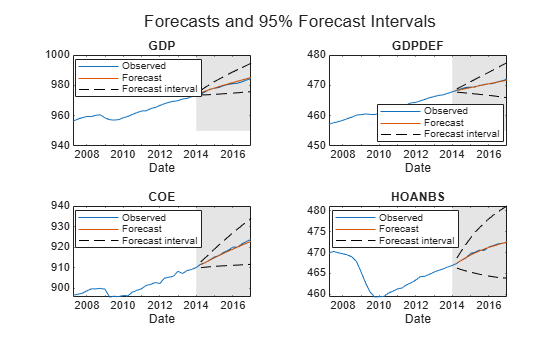figure;
subplot(2,2,1)
h1 = plot(FRED.Time((end-39):end),FRED.FEDFUNDS((end-39):end));
hold on
h2 = plot(FRED.Time(~estIdx),Y(:,5));
h3 = plot(FRED.Time(~estIdx),YFI(:,5,1),'k--');
plot(FRED.Time(~estIdx),YFI(:,5,2),'k--');
title('Federal Funds Rate');
ylabel('Percent');
xlabel('Date');
h = gca;
fill([bfh h.XLim([2 2]) bfh],h.YLim([1 1 2 2]),'k',...
'FaceAlpha',0.1,'EdgeColor','none');
legend([h1 h2 h3],'True','Forecast','95% Forecast interval',...
'Location','best');
hold off
subplot(2,2,2)
h1 = plot(FRED.Time((end-39):end),FRED.PCEC((end-39):end));
hold on
h2 = plot(FRED.Time(~estIdx),Y(:,6));
h3 = plot(FRED.Time(~estIdx),YFI(:,6,1),'k--');
plot(FRED.Time(~estIdx),YFI(:,6,2),'k--');
title('Consumption Expenditures');
ylabel('Billions of \$ (scaled)');
xlabel('Date');
h = gca;
fill([bfh h.XLim([2 2]) bfh],h.YLim([1 1 2 2]),'k',...
'FaceAlpha',0.1,'EdgeColor','none');
legend([h1 h2 h3],'True','Forecast','95% Forecast interval',...
'Location','best');
hold off
subplot(2,2,3)
h1 = plot(FRED.Time((end-39):end),FRED.GPDI((end-39):end));
hold on
h2 = plot(FRED.Time(~estIdx),Y(:,7));
h3 = plot(FRED.Time(~estIdx),YFI(:,7,1),'k--');
plot(FRED.Time(~estIdx),YFI(:,7,2),'k--');
title('Gross Private Domestic Investment');
ylabel('Billions of \$ (scaled)');
xlabel('Date');
h = gca;
fill([bfh h.XLim([2 2]) bfh],h.YLim([1 1 2 2]),'k',...
'FaceAlpha',0.1,'EdgeColor','none');
legend([h1 h2 h3],'True','Forecast','95% Forecast interval',...
'Location','best');
hold off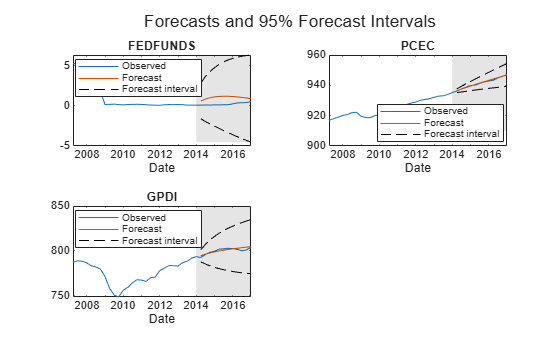Input Arguments

collapse all

VEC model, specified as a vecm model object created by vecm or estimate. Mdl must be fully specified.

Forecast horizon, or the number of time points in the forecast period, specified as a positive integer.

Data Types: double

Presample responses that provide initial values for the forecasts, specified as a numpreobs-by-numseries numeric matrix or a numpreobs-by-numseries-by-numprepaths numeric array.

numpreobs is the number of presample observations. numseries is the number of response series (Mdl.NumSeries). numprepaths is the number of presample response paths.

Rows correspond to presample observations, and the last row contains the latest observation. Y0 must contain at least Mdl.P rows. If you supply more rows than necessary, forecast uses only the latest Mdl.P observations.

Columns must correspond to the response series names in Mdl.SeriesNames.

Pages correspond to separate, independent paths.

• If you do not specify the YF name-value pair argument, then forecast initializes each forecasted path (page) using the corresponding page of Y0. Therefore, the output argument Y has numprepaths pages.

• If you specify the YF name-value pair argument, then forecast takes one of these actions.

• If Y0 is a matrix, then forecast initializes each forecast path (page) in YF using Y0. Therefore, all paths in the output argument Y derive from common initial conditions.

• Otherwise, forecast applies Y0(:,:,j) to initialize forecasting path j. Y0 must have at least numpaths pages, and forecast uses only the first numpaths pages.

Among all pages, observations in a particular row occur at the same time.

Data Types: double

Name-Value Arguments

Specify optional comma-separated pairs of Name,Value arguments. Name is the argument name and Value is the corresponding value. Name must appear inside quotes. You can specify several name and value pair arguments in any order as Name1,Value1,...,NameN,ValueN.

Example: 'X',X,'YF',YF uses the matrix X as predictor data in the regression component, and the matrix YF as partially known future responses for conditional forecasting.

Forecasted time series of predictors to include in the model regression component, specified as the comma-separated pair consisting of 'X' and a numeric matrix containing numpreds columns.

numpreds is the number of predictor variables (size(Mdl.Beta,2)).

Rows correspond to observations. Row j contains the j-step-ahead forecast. X must have at least numperiods rows. If you supply more rows than necessary, forecast uses only the earliest numperiods observations. The first row contains the earliest observation.

Columns correspond to individual predictor variables. All predictor variables are present in the regression component of each response equation.

forecast applies X to each path (page); that is, X represents one path of observed predictors.

To maintain model consistency into the forecast horizon, it is a good practice to specify forecasted predictors when Mdl has a regression component.

By default, forecast excludes the regression component, regardless of its presence in Mdl.

Data Types: double

Future multivariate response series for conditional forecasting, specified as the comma-separated pair consisting of 'YF' and a numeric matrix or 3-D array containing numseries columns.

Rows correspond to observations in the forecast horizon, and the first row is the earliest observation. Specifically, row j in sample path k (YF(j,:,k)) contains the responses j periods into the future. YF must have at least numperiods rows to cover the forecast horizon. If you supply more rows than necessary, forecast uses only the first numperiods rows.

Columns correspond to the response variables in Y0.

Pages correspond to sample paths. Specifically, path k (YF(:,:,k)) captures the state, or knowledge, of the response series as they evolve from the presample past (Y0) into the future.

• If YF is a matrix, then forecast applies YF to each of the numpaths output paths (see Y0).

• Otherwise, YF must have at least numpaths pages. If you supply more pages than necessary, forecast uses only the first numpaths pages.

Elements of YF can be numeric scalars or missing values (indicated by NaN values). forecast treats numeric scalars as deterministic future responses that are known in advance, for example, set by policy. forecast forecasts responses for corresponding NaN values conditional on the known values.

By default, YF is an array composed of NaN values indicating a complete lack of knowledge of the future state of all responses in the forecast horizon. In this case, forecast estimates conventional MMSE forecasts.

For more details, see Algorithms.

Example: Consider forecasting one path of a VEC model composed of four response series three periods into the future. Suppose that you have prior knowledge about some of the future values of the responses, and you want to forecast the unknown responses conditional on your knowledge. Specify YF as a matrix containing the values that you know, and use NaN for values you do not know but want to forecast. For example, 'YF',[NaN 2 5 NaN; NaN NaN 0.1 NaN; NaN NaN NaN NaN] specifies that you have no knowledge of the future values of the first and fourth response series; you know the value for period 1 in the second response series, but no other value; and you know the values for periods 1 and 2 in the third response series, but not the value for period 3.

Data Types: double

Note

NaN values in Y0 and X indicate missing values. forecast removes missing values from the data by list-wise deletion. If Y0 is a 3-D array, then forecast performs these steps.

1. Horizontally concatenate pages to form a numpreobs-by-numpaths*numseries matrix.

2. Remove any row that contains at least one NaN from the concatenated data.

In the case of missing observations, the results obtained from multiple paths of Y0 can differ from the results obtained from each path individually.

For missing values in X, forecast removes the corresponding row from each page of YF. After row removal from X and YF, if the number of rows is less than numperiods, then forecast throws an error.

Output Arguments

collapse all

MMSE forecasts of the multivariate response series, returned as a numobs-by-numseries numeric matrix or a numobs-by-numseries-by-numpaths numeric array. Y represents the continuation of the presample responses in Y0. Rows correspond to observations, columns correspond to response variables, and pages correspond to sample paths. Row j is the j-period-ahead forecast.

If you specify future responses for conditional forecasting using the YF name-value pair argument, then the known values in YF appear in the same positions in Y. However, Y contains forecasted values for the missing observations in YF.

MSE matrices of the forecasted responses in Y, returned as a numperiods-by-1 cell vector of numseries-by-numseries numeric matrices. Cells of YMSE compose a time series of forecast error covariance matrices. Cell j contains the j-period-ahead MSE matrix.

YMSE is identical for all paths.

Because forecast treats predictor variables in X as exogenous and non-stochastic, YMSE reflects the error covariance associated with the autoregressive component of the input model Mdl only.

Algorithms

• forecast estimates unconditional forecasts using the equation

$\Delta {\stackrel{^}{y}}_{t}=\stackrel{^}{A}{\stackrel{^}{B}}^{\prime }{\stackrel{^}{y}}_{t-1}+{\stackrel{^}{\Phi }}_{1}\Delta {\stackrel{^}{y}}_{t-1}+...+{\stackrel{^}{\Phi }}_{p}\Delta {\stackrel{^}{y}}_{t-p}+\stackrel{^}{c}+\stackrel{^}{d}t+{x}_{t}\stackrel{^}{\beta },$

where t = 1,...,numperiods. forecast filters a numperiods-by-numseries matrix of zero-valued innovations through Mdl. forecast uses specified presample innovations (Y0) wherever necessary.

• forecast estimates conditional forecasts using the Kalman filter.

1. forecast represents the VEC model Mdl as a state-space model (ssm model object) without observation error.

2. forecast filters the forecast data YF through the state-space model. At period t in the forecast horizon, any unknown response is

$\Delta {\stackrel{^}{y}}_{t}=\stackrel{^}{A}{\stackrel{^}{B}}^{\prime }{\stackrel{^}{y}}_{t-1}+{\stackrel{^}{\Phi }}_{1}\Delta {\stackrel{^}{y}}_{t-1}+...+{\stackrel{^}{\Phi }}_{p}\Delta {\stackrel{^}{y}}_{t-p}+\stackrel{^}{c}+\stackrel{^}{d}t+{x}_{t}\stackrel{^}{\beta },$

where ${\stackrel{^}{y}}_{s},$ s < t, is the filtered estimate of y from period s in the forecast horizon. forecast uses specified presample values in Y0 for periods before the forecast horizon.

For more details, see filter and , pp. 612 and 615.

• The way forecast determines numpaths, the number of pages in the output argument Y, depends on the forecast type.

• If you estimate unconditional forecasts, which means you do not specify the name-value pair argument YF, then numpaths is the number of pages in the input argument Y0.

• If you estimate conditional forecasts and Y0 and YF have more than one page, then numpaths is the number of pages in the array with fewer pages. If the number of pages in Y0 or YF exceeds numpaths, then forecast uses only the first numpaths pages.

• If you estimate conditional forecasts and either Y0 or YF has one page, then numpaths is the number of pages in the array with the most pages. forecast uses the array with one page for each path.

• forecast sets the time origin of models that include linear time trends (t0) to size(Y0,1)Mdl.P (after removing missing values). Therefore, the times in the trend component are t = t0 + 1, t0 + 2,..., t0 + numobs. This convention is consistent with the default behavior of model estimation in which estimate removes the first Mdl.P responses, reducing the effective sample size. Although forecast explicitly uses the first Mdl.P presample responses in Y0 to initialize the model, the total number of observations (excluding missing values) determines t0.

 Hamilton, James D. Time Series Analysis. Princeton, NJ: Princeton University Press, 1994.

 Johansen, S. Likelihood-Based Inference in Cointegrated Vector Autoregressive Models. Oxford: Oxford University Press, 1995.

 Juselius, K. The Cointegrated VAR Model. Oxford: Oxford University Press, 2006.

 Lütkepohl, H. New Introduction to Multiple Time Series Analysis. Berlin: Springer, 2005.